Computational & Technology Resources
an online resource for computational,
engineering & technology publications
Civil-Comp Proceedings
ISSN 1759-3433
CCP: 73
PROCEEDINGS OF THE EIGHTH INTERNATIONAL CONFERENCE ON CIVIL AND STRUCTURAL ENGINEERING COMPUTING
Edited by: B.H.V. Topping
Paper 57

Optimum Design of Reinforced Concrete Continuous Beams by Genetic Algorithms

Faculty of Engineering, University of Wollongong, Australia

Full Bibliographic Reference for this paper
M.N.S. Hadi, "Optimum Design of Reinforced Concrete Continuous Beams by Genetic Algorithms", in B.H.V. Topping, (Editor), "Proceedings of the Eighth International Conference on Civil and Structural Engineering Computing", Civil-Comp Press, Stirlingshire, UK, Paper 57, 2001. doi:10.4203/ccp.73.57
Keywords: genetic algorithm, reinforced concrete, beams, optimum design.

Summary
This paper presents the application of Genetic Algorithms for the design of continuous reinforced concrete T and L beams based on the recommendations of the current Australian design standards. This optimisation problem was implemented by writing a computer program using MATLAB. This computer program is composed of two modules, one for structural analysis and design of the beams and the second for calculating their optimum solution using the genetic algorithm code. A number of examples were analysed and designed using the developed code and proved that the produced design is economical, also it proved that developed program is efficient and versatile.

The design of statically indeterminate structures requires the assumption of members' dimensions, followed by analysis of the whole structure, then followed by the design of the members. At the end of this design process, a comparison is made between the initial design and the produced design. If the assumed initial design was smaller than the produced design, then a redesign is necessary. However, if the assumed design was larger than the produced design, then it is up to the designer to make the decision of whether to adopt the initial design or to start again the design process. Each phase of the design/analysis/design cycle is usually lengthy and requires a considerable amount of time and effort. The designer should repeat the process several times in order to obtain a structure that is both safe and economical.

In order to reduce the time and effort involved in the design of economical structures, methods of optimisation have been successfully applied for the last three decades. The success of these methods has been considerable and led to savings both in construction materials and during the design process. However, most such optimisation techniques involve a considerable amount of mathematical formulation and computer programming. In this paper, the optimum design of structures is sought using genetic algorithms. As such, a computer program using Matlab for the optimum design of continuous T and L beams based on genetic algorithms has been developed. The choice of T and L beams was made, as they are not only demanding in analysis but also require a considerable amount of time in design. The problem of optimum design of continuous T and L beams was formulated based on the requirements of the current Australian Standards and the stiffness method is used to analyse the continuous beam. The objective is to minimise the total cost of the beam.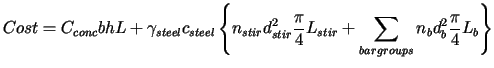(57.1)

where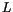= beam length;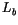= length of bars in bar group;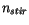= number of stirrups;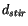= stirrup diameter;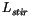= length of a single stirrup;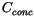= concrete cost per volume;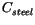= steel cost per weight;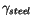= unit weight of steel; and= diameter of bar.

Constraints are imposed according to the Australian Standard, AS3600  at each critical location: locations with maximum positive bending moment; negative bending moment and maximum shear force. The following constraints are imposed at each positive bending moment and negative bending moment critical location.

 Flexural strength: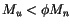Minimum reinforcement ratio: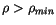Spacing: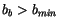where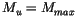(positive location) or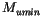(negative location);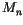= flexural strength;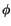= strength reduction factor;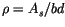;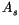= total reinforcement area at the location;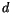= distance from extreme concrete compressive fibre to reinforcement centroid;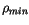= 1.4 MPa /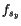; and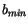= smallest dimensions with bars at minimum. Critical locations for shear strength are located at a distancefrom the face of each support. Shear strength may be supplemented by the addition of stirrups at critical shear locations unless the following constant is violated.
 Shear strength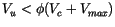where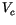= concrete shear strength;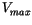= maximum allowable stirrup shear strength; and= strength reduction factor. The evaluation conducted in this study, proved that GA achieves the goal of optimisation of T and L beams quickly and without the need to use high level of mathematics. Finally, as GA is a versatile concept, it can be applied for the design of other structural members easily.

References
1
Australian Standard, "Concrete Structures", Standards Australia, AS 3600, 1994.

purchase the full-text of this paper (price £20)